# Physical Chemistry : Calorimetry

## Example Questions

### Example Question #21 : Physical Chemistry

The heat capacity of a bomb calorimeter assembly is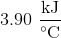. What is the heat of combustion of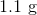of sucrose in a bomb calorimeter that results in the temperature rising from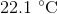to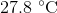?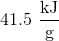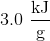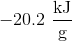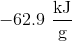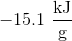Explanation:

A bomb calorimeter is a device used to measure the quantity of heat change for a process. The heat of a reaction which is denoted as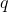, is the negative of the thermal energy gained by the calorimeter: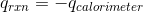The heat capacity of a calorimeter is: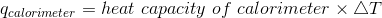Plugging the values given into the equation gives: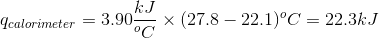Using the relation provided earlier: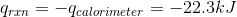Because we are dealing with 1.1 grams of sucrose, the heat of combustion of sucrose is: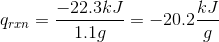### Example Question #21 : Physical Chemistry

The heat capacity of a bomb calorimeter assembly is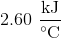. What is the heat of combustion of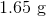of caffeine in a bomb calorimeter that results in the temperature rising from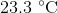to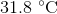?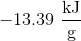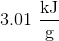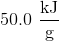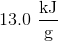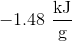Explanation:

A bomb calorimeter is a device used to measure the quantity of heat change for a process. The heat of a reaction which is denoted as q, is the negative of the thermal energy gained by the calorimeter:The heat capacity of a calorimeter is:Plugging the values given into the equation gives: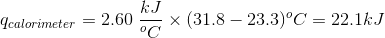Using the relation provided earlier: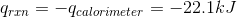Because we are dealing with 1.65 grams of sucrose, the heat of combustion of sucrose is: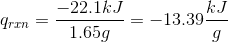### All Physical Chemistry Resources